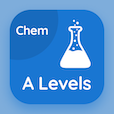Cambridge Online Courses (GCSE)

O Level Physics Quizzes

O Level Physics Quiz PDF - Complete

# Latent Heat Quiz Questions Online p. 97

Learn Latent Heat quiz questions and answers, latent heat MCQ with answers PDF 97 to study O Level Physics course online. Thermal Properties of Matter trivia questions, latent heat Multiple Choice Questions (MCQ) for online college degrees. "Latent Heat Quiz" PDF Book: scalar and vector, inertia, mass and weight, temperature scales, physical quantities and si unit, latent heat test prep to learn online certificate courses.

"Boiling is" MCQ PDF: quicker than evaporation, slower than evaporation, same as evaporation, and totally different thing for online college for teaching degree. Study thermal properties of matter questions and answers to improve problem solving skills for online colleges for science.

## Quiz on Latent Heat MCQs

MCQ: Boiling is

slower than evaporation
quicker than evaporation
same as evaporation
totally different thing

MCQ: A physical quantity consists of a

Analogical Magnitude
Numerical magnitude
Alphabetical Magnitude
Symbolic Magnitude

MCQ: A thermometer that is alcohol-in-glass thermometer has l100 23 cm and l0 is 8 cm. If the lθ is 2 cm the temperature of the thermometer would be

−10 °C
−20 °C
−30 °C
−40 °C

MCQ: For an object of mass 4 kg, the incorrect statement is

Weight of the object on earth = 10 × Mass of the object on earth
Mass of the object on earth = Mass of the object on moon
Weight of the object on earth = 1 ⁄ 6 × Weight of the object on moon
Weight of the object on moon = 1 ⁄ 6 × Weight of the object on earth

MCQ: Two forces of magnitude 5 N and 10 N act on a wooden block of mass 2 kg. If 5 N force acts towards right and 10 N force acts towards left, which of the following statements is correct?

Resultant force is 15 N towards the left
Resultant force is 15 N towards the right
Resultant force is 5 N towards the right
Resultant force is 5N towards the left

### More Quizzes from O Level Physics Course